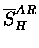Next: Relative acceptance Up: Corrections to in the Previous: Charged particles from muon

### Electron energy spectrum

As was seen in the previous section, the effect of capture induced charged particles alone cannot explain the energy dependence of. Indeed, for the downstream counters, it was found very unlikely to cause any measurable effect. We therefore turn our attention to other effects in these two sections, i.e., electron energy spectra, and upstream-downstream acceptance difference.

The energy spectrum of decay electrons from muons bound in a nucleus is known to differ from that in free space, due to effects such as the final state Coulomb interaction and reduced phase space [226,227,228] (the same effects are responsible for the Huff factor describing the reduction in decay rates). Shown in Fig. 6.7 are the electron energy spectra calculated by Huff , for muons bound in lead and iron, together with that for free muon decay. As all the spectra are normalized to a free muon decay rate of unity, the integral of the spectra gives the Huff factor (Q=0.844for lead, 0.975 for iron). More recent and more elaborate calculations on the energy spectra [227,228] essentially agree with Huff's.

Figure 6.7 illustrates that the strength of the spectra above MeV, energies which would give a peak in the telescope spectrum (Fig. 6.6), is significantly larger for free decay than for lead (which are similar to decay for hydrogen and for gold, respectively). This would cause the amplitude ratio for hydrogento increase at higher energies. At lower energies, on the other hand, the spectrum is stronger for lead than for free decay, which would decrease. Thus the difference in the electron energy spectra shown in Fig. 6.7 provides an explanation, at least in part, for the energy dependence of the amplitude ratioobserved in Fig. 6.6.Next: Relative acceptance Up: Corrections to in the Previous: Charged particles from muon